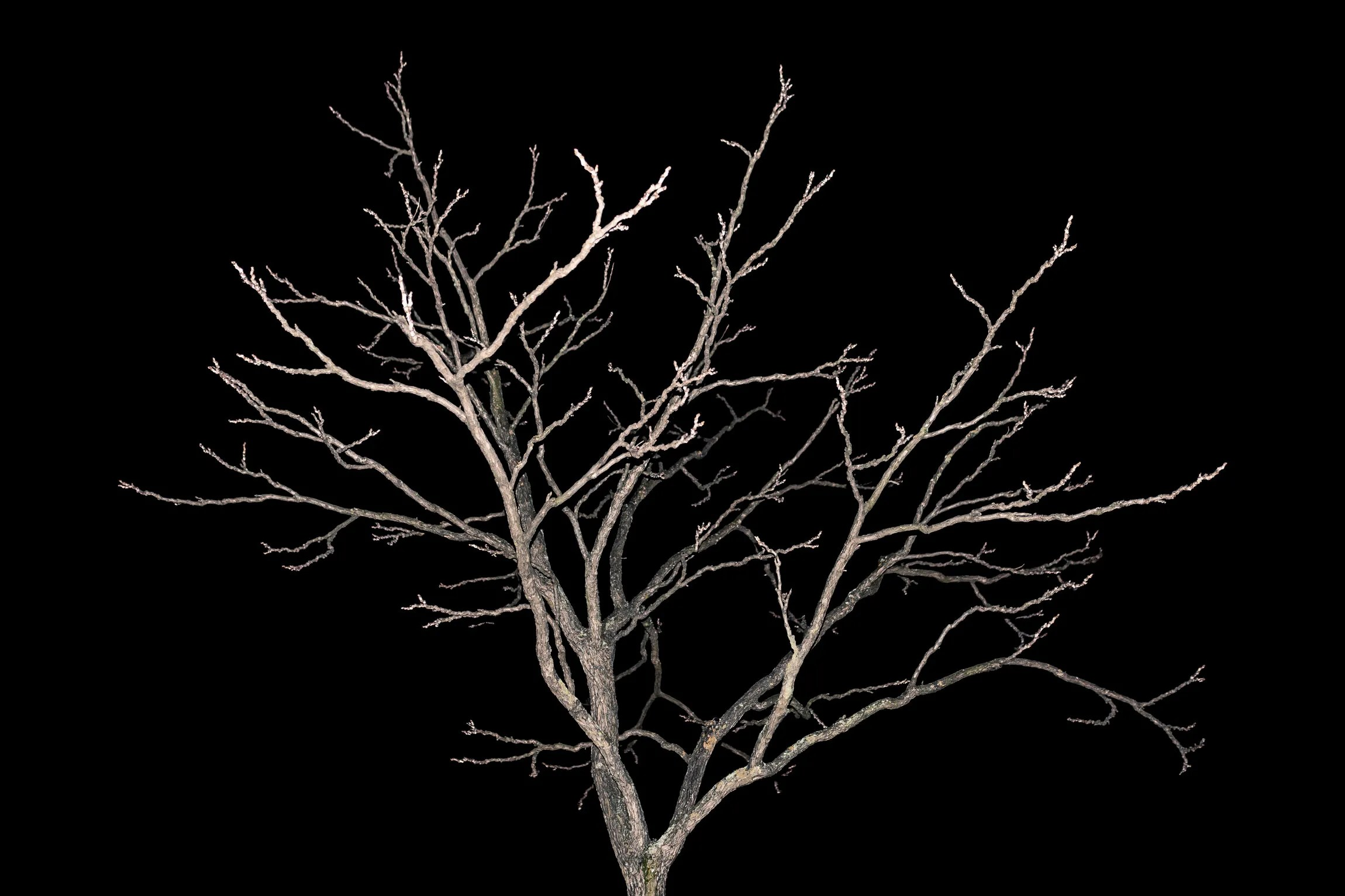# How to Make a Tree With FractalsAnway, the thing to notice here is how it works: I created a function called “pfact” to perform the operations, and the definition of the function actually calls itself (in line 11 ). That’s recursion. By creating that nested structure, we can do elaborate calculations using very little code. It’s sort of amazing that this works.

Recursion in the Wild

So what about fractals? Fractals are patterns that have the same appearance at different scales. You find them everywhere in nature. Look at a coastline: It’s full of bays and rivers and peninsulas. If you zoom in on a small section, is it smoother? Nope, up close you see the same jagged shapes at smaller scale.

This self-similarity can also be seen in trees. If you start from the trunk and move up, it breaks into several parts—we call these branches. If you follow one of these branches, it too splits in a way that is similar to the previous branch. Each branch of the tree is itself a smaller tree shape. So a tree is like a fractal. That means we can model a tree using fractals.

I think we’re ready for a tree fractal. I’m going to show you how to make this fractal with GlowScript Python. Of course there are other options. Perhaps you prefer to do it with Python and Turtle? Here’s a nice tutorial for that.

Here is the basic plan for this tree fractal:

• Start at some point and move a certain distance in a certain direction.
• At that point, make a branch. Turn some angle to the right and then repeat the previous step with a shorter distance. (Recursion!)
• Now go back and turn left to make the other branch. (Recursion again.)

You probably won’t really understand this code until you break it. So here you go—this is my first tree fractal. Run this, and then change some stuff. If you click the pencil icon, you can see the code and edit it.

A quick note about vectors and cylinders. Since each branch is a cylinder in three dimensions, there are some parts of the code that might be confusing. When you make a cylinder in GlowScript Python, you need two things: a starting position (a vector in 3D space) and another vector that points from the start to the end of the cylinder. In the code, this pointing vector is the variable a—it’s this vector that gets rotated in each branching.

Branching Out

Enough about vectors—let’s make some cool stuff! What if I want to make my tree more tree-like? Here are some things I can change: